Author: Oscar Cronquist Article last updated on June 07, 2022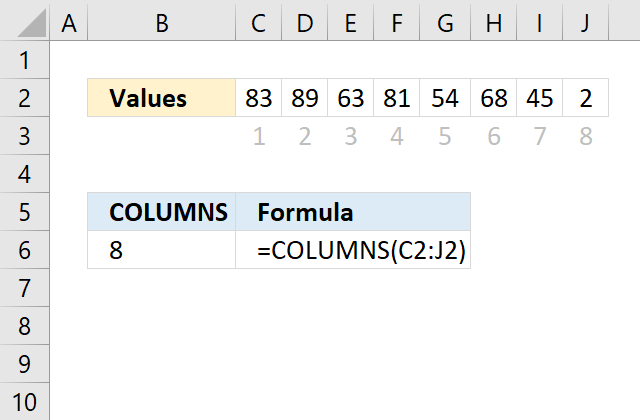The COLUMNS function allows you to calculate the number of columns in a cell range.

COLUMNS(array)

## 2. COLUMNS Function Arguments

 array Required. A cell range for which you want to determine the number of columns.

## 3. COLUMNS Function exampleThe example above shows that cell range C2:J2 has 8 columns.

Formula in cell B6:

=COLUMNS(C2:J2)

## 4. Count columns in an array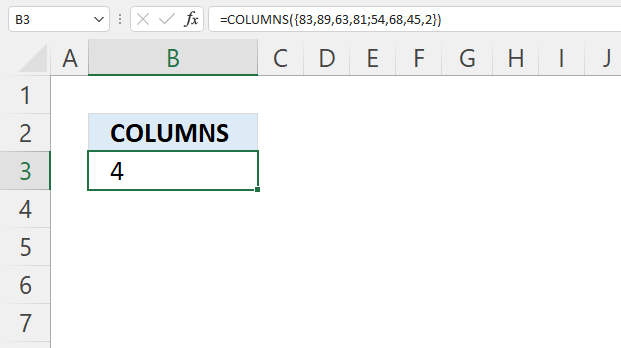The COLUMNS function also calculates the number of rows in an array.

=COLUMNS({83,89,63,81;54,68,45,2})

The array has four columns. An array uses delimiting values to separate columns and rows.  They may differ between users based on their regional settings.

The formula above uses comma as a column delimiting character and semicolon as a row delimiting character.

83,89,63,81;
54,68,45,2

It is easier to see that the array actually contains four columns if I put values after a semicolon on a new row.

## 5. Count columns in a string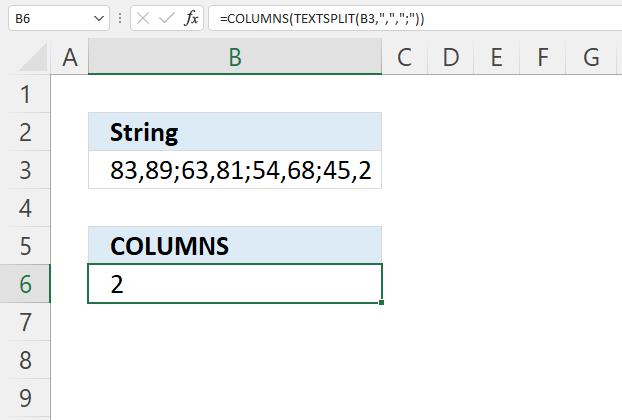Formula in cell B6:

=COLUMNS(TEXTSPLIT(B3,",",";"))

### 5.1 Explaining formula

#### Step 1 - Split string into an array

TEXTSPLIT(B3,",",";")

#### Step 2 - Count columns in the array

COLUMNS(TEXTSPLIT(B3,",",";"))

## 6. Count columns in multiple cell ranges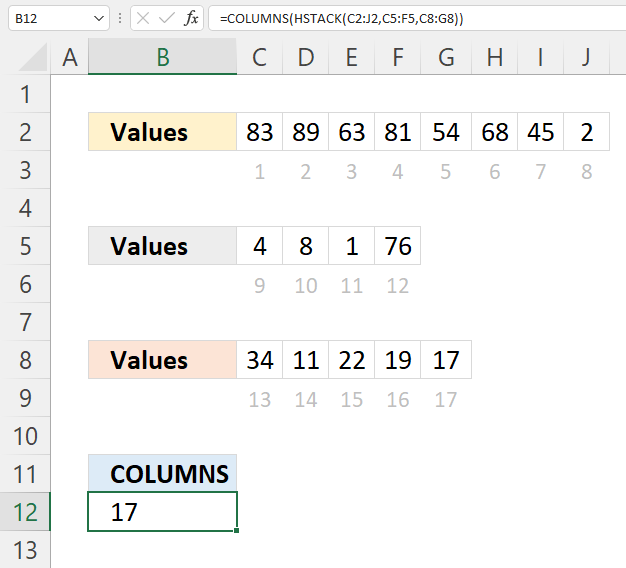Formula in cell B12:

=COLUMNS(HSTACK(C2:J2,C5:F5,C8:G8))

### 6.1 Explaining formula

#### Step 1 - Join cell ranges horizontally

HSTACK(C2:J2,C5:F5,C8:G8)

#### Step 2 - Count columns

COLUMNS(HSTACK(C2:J2,C5:F5,C8:G8))

## 7. Count columns in multiple Excel Tables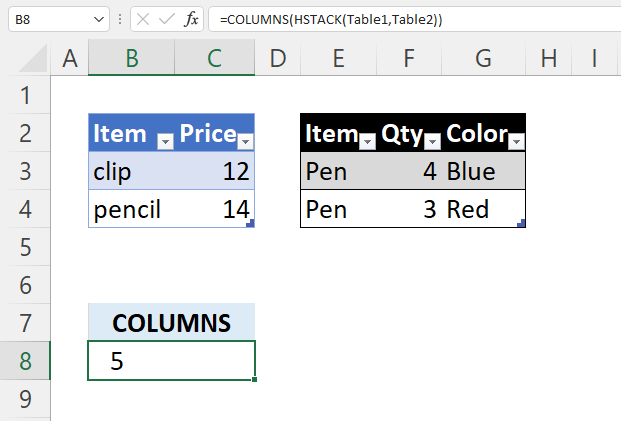Formula in cell B8:

=COLUMNS(HSTACK(Table1, Table2))

### 7.1 Explaining formula

#### Step 1 - Join Excel Tables horizontally

HSTACK(Table1, Table2)

#### Step 2 - Count columns

COLUMNS(HSTACK(Table1, Table2))